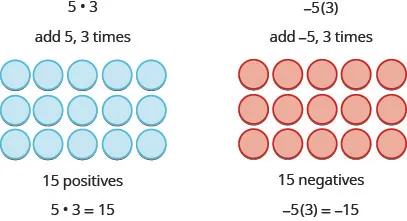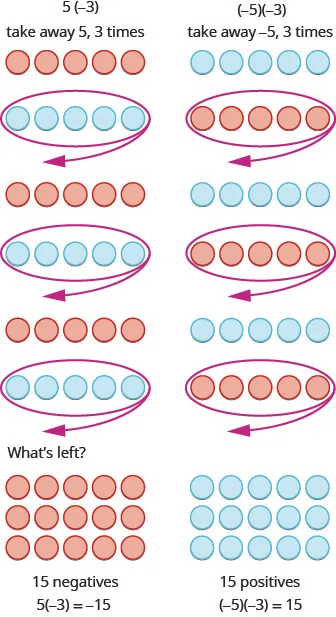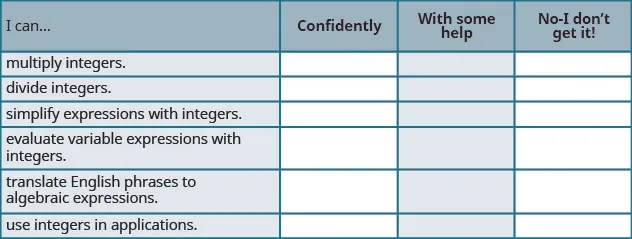Elementary Algebra 2e

# 1.4Multiply and Divide Integers

Elementary Algebra 2e1.4 Multiply and Divide Integers

### Learning Objectives

By the end of this section, you will be able to:

• Multiply integers
• Divide integers
• Simplify expressions with integers
• Evaluate variable expressions with integers
• Translate English phrases to algebraic expressions
• Use integers in applications

### Be Prepared 1.4

A more thorough introduction to the topics covered in this section can be found in the Prealgebra chapter, Integers.

### Multiply Integers

Since multiplication is mathematical shorthand for repeated addition, our model can easily be applied to show multiplication of integers. Let’s look at this concrete model to see what patterns we notice. We will use the same examples that we used for addition and subtraction. Here, we will use the model just to help us discover the pattern.

We remember that $a·ba·b$ means add a, b times.The next two examples are more interesting.

What does it mean to multiply 5 by $−3?−3?$ It means subtract 5, 3 times. Looking at subtraction as “taking away,” it means to take away 5, 3 times. But there is nothing to take away, so we start by adding neutral pairs on the workspace. Then we take away 5 three times.In summary:

$5·3=15−5(3)=−155(−3)=−15(−5)(−3)=155·3=15−5(3)=−155(−3)=−15(−5)(−3)=15$

Notice that for multiplication of two signed numbers, when the:

• signs are the same, the product is positive.
• signs are different, the product is negative.

We’ll put this all together in the chart below.

### Multiplication of Signed Numbers

For multiplication of two signed numbers:

Same signs Product Example
Two positives
Two negatives
Positive
Positive
$7·4=28−8(−6)=487·4=28−8(−6)=48$
Different signs Product Example
Positive · negative
Negative · positive
Negative
Negative
$7(−9)=−63−5·10=−507(−9)=−63−5·10=−50$

### Example 1.46

Multiply: $−9·3−9·3$ $−2(−5)−2(−5)$ $4(−8)4(−8)$ $7·6.7·6.$

### Try It 1.91

Multiply: $−6·8−6·8$ $−4(−7)−4(−7)$ $9(−7)9(−7)$ $5·12.5·12.$

### Try It 1.92

Multiply: $−8·7−8·7$ $−6(−9)−6(−9)$ $7(−4)7(−4)$ $3·13.3·13.$

When we multiply a number by 1, the result is the same number. What happens when we multiply a number by $−1?−1?$ Let’s multiply a positive number and then a negative number by $−1−1$ to see what we get.

$−1·4−1(−3)Multiply.−43−4is the opposite of4.3is the opposite of−3.−1·4−1(−3)Multiply.−43−4is the opposite of4.3is the opposite of−3.$

Each time we multiply a number by $−1,−1,$ we get its opposite!

### Multiplication by $−1 −1$

$−1a=−a−1a=−a$

Multiplying a number by $−1−1$ gives its opposite.

### Example 1.47

Multiply: $−1·7−1·7$ $−1(−11).−1(−11).$

### Try It 1.93

Multiply: $−1·9−1·9$ $−1·(−17).−1·(−17).$

### Try It 1.94

Multiply: $−1·8−1·8$ $−1·(−16).−1·(−16).$

### Divide Integers

What about division? Division is the inverse operation of multiplication. So, $15÷3=515÷3=5$ because $5·3=15.5·3=15.$ In words, this expression says that 15 can be divided into three groups of five each because adding five three times gives 15. Look at some examples of multiplying integers, to figure out the rules for dividing integers.

$5·3=15so15÷3=5−5(3)=−15so−15÷3=−5(−5)(−3)=15so15÷(−3)=−55(−3)=−15so−15÷(−3)=55·3=15so15÷3=5−5(3)=−15so−15÷3=−5(−5)(−3)=15so15÷(−3)=−55(−3)=−15so−15÷(−3)=5$

Division follows the same rules as multiplication!

For division of two signed numbers, when the:

• signs are the same, the quotient is positive.
• signs are different, the quotient is negative.

And remember that we can always check the answer of a division problem by multiplying.

### Multiplication and Division of Signed Numbers

For multiplication and division of two signed numbers:

• If the signs are the same, the result is positive.
• If the signs are different, the result is negative.
Same signs Result
Two positives
Two negatives
Positive
Positive
If the signs are the same, the result is positive.
Different signs Result
Positive and negative
Negative and positive
Negative
Negative
If the signs are different, the result is negative.

### Example 1.48

Divide: $−27÷3−27÷3$ $−100÷(−4).−100÷(−4).$

### Try It 1.95

Divide: $−42÷6−42÷6$ $−117÷(−3).−117÷(−3).$

### Try It 1.96

Divide: $−63÷7−63÷7$ $−115÷(−5).−115÷(−5).$

### Simplify Expressions with Integers

What happens when there are more than two numbers in an expression? The order of operations still applies when negatives are included. Remember My Dear Aunt Sally?

Let’s try some examples. We’ll simplify expressions that use all four operations with integers—addition, subtraction, multiplication, and division. Remember to follow the order of operations.

### Example 1.49

Simplify: $7(−2)+4(−7)−6.7(−2)+4(−7)−6.$

### Try It 1.97

Simplify: $8(−3)+5(−7)−4.8(−3)+5(−7)−4.$

### Try It 1.98

Simplify: $9(−3)+7(−8)−1.9(−3)+7(−8)−1.$

### Example 1.50

Simplify: $(−2)4(−2)4$ $−24.−24.$

### Try It 1.99

Simplify: $(−3)4(−3)4$ $−34.−34.$

### Try It 1.100

Simplify: $(−7)2(−7)2$ $−72.−72.$

The next example reminds us to simplify inside parentheses first.

### Example 1.51

Simplify: $12−3(9−12).12−3(9−12).$

### Try It 1.101

Simplify: $17−4(8−11).17−4(8−11).$

### Try It 1.102

Simplify: $16−6(7−13).16−6(7−13).$

### Example 1.52

Simplify: $8(−9)÷(−2)3.8(−9)÷(−2)3.$

### Try It 1.103

Simplify: $12(−9)÷(−3)3.12(−9)÷(−3)3.$

### Try It 1.104

Simplify: $18(−4)÷(−2)3.18(−4)÷(−2)3.$

### Example 1.53

Simplify: $−30÷2+(−3)(−7).−30÷2+(−3)(−7).$

### Try It 1.105

Simplify: $−27÷3+(−5)(−6).−27÷3+(−5)(−6).$

### Try It 1.106

Simplify: $−32÷4+(−2)(−7).−32÷4+(−2)(−7).$

### Evaluate Variable Expressions with Integers

Remember that to evaluate an expression means to substitute a number for the variable in the expression. Now we can use negative numbers as well as positive numbers.

### Example 1.54

When $n=−5,n=−5,$ evaluate: $n+1n+1$ $−n+1.−n+1.$

### Try It 1.107

When $n=−8,n=−8,$ evaluate $n+2n+2$ $−n+2.−n+2.$

### Try It 1.108

When $y=−9,y=−9,$ evaluate $y+8y+8$ $−y+8.−y+8.$

### Example 1.55

Evaluate $(x+y)2(x+y)2$ when $x=−18x=−18$ and $y=24.y=24.$

### Try It 1.109

Evaluate $(x+y)2(x+y)2$ when $x=−15x=−15$ and $y=29.y=29.$

### Try It 1.110

Evaluate $(x+y)3(x+y)3$ when $x=−8x=−8$ and $y=10.y=10.$

### Example 1.56

Evaluate $20−z20−z$ when $z=12z=12$ and $z=−12.z=−12.$

### Try It 1.111

Evaluate: $17−k17−k$ when $k=19k=19$ and $k=−19.k=−19.$

### Try It 1.112

Evaluate: $−5−b−5−b$ when $b=14b=14$ and $b=−14.b=−14.$

### Example 1.57

Evaluate: $2x2+3x+82x2+3x+8$ when $x=4.x=4.$

### Try It 1.113

Evaluate: $3x2−2x+63x2−2x+6$ when $x=−3.x=−3.$

### Try It 1.114

Evaluate: $4x2−x−54x2−x−5$ when $x=−2.x=−2.$

### Translate Phrases to Expressions with Integers

Our earlier work translating English to algebra also applies to phrases that include both positive and negative numbers.

### Example 1.58

Translate and simplify: the sum of 8 and $−12,−12,$ increased by 3.

### Try It 1.115

Translate and simplify the sum of 9 and $−16,−16,$ increased by 4.

### Try It 1.116

Translate and simplify the sum of $−8−8$ and $−12,−12,$ increased by 7.

When we first introduced the operation symbols, we saw that the expression may be read in several ways. They are listed in the chart below.

$a−ba−b$
$aa$ minus $bb$
the difference of $aa$ and $bb$
$bb$ subtracted from $aa$
$bb$ less than $aa$

Be careful to get a and b in the right order!

### Example 1.59

Translate and then simplify the difference of 13 and $−21−21$ subtract 24 from $−19.−19.$

### Try It 1.117

Translate and simplify the difference of 14 and $−23−23$ subtract 21 from $−17.−17.$

### Try It 1.118

Translate and simplify the difference of 11 and $−19−19$ subtract 18 from $−11.−11.$

Once again, our prior work translating English to algebra transfers to phrases that include both multiplying and dividing integers. Remember that the key word for multiplication is “product” and for division is “quotient.”

### Example 1.60

Translate to an algebraic expression and simplify if possible: the product of $−2−2$ and 14.

### Try It 1.119

Translate to an algebraic expression and simplify if possible: the product of $−5−5$ and 12.

### Try It 1.120

Translate to an algebraic expression and simplify if possible: the product of 8 and $−13.−13.$

### Example 1.61

Translate to an algebraic expression and simplify if possible: the quotient of $−56−56$ and $−7.−7.$

### Try It 1.121

Translate to an algebraic expression and simplify if possible: the quotient of $−63−63$ and $−9.−9.$

### Try It 1.122

Translate to an algebraic expression and simplify if possible: the quotient of $−72−72$ and $−9.−9.$

### Use Integers in Applications

We’ll outline a plan to solve applications. It’s hard to find something if we don’t know what we’re looking for or what to call it! So when we solve an application, we first need to determine what the problem is asking us to find. Then we’ll write a phrase that gives the information to find it. We’ll translate the phrase into an expression and then simplify the expression to get the answer. Finally, we summarize the answer in a sentence to make sure it makes sense.

### Example 1.62

#### How to Apply a Strategy to Solve Applications with Integers

In the morning, the temperature in Urbana, Illinois was 11 degrees. By mid-afternoon, the temperature had dropped to $−9−9$ degrees. What was the difference of the morning and afternoon temperatures?

### Try It 1.123

In the morning, the temperature in Anchorage, Alaska was 15 degrees. By mid-afternoon the temperature had dropped to 30 degrees below zero. What was the difference in the morning and afternoon temperatures?

### Try It 1.124

The temperature in Denver was $−6−6$ degrees at lunchtime. By sunset the temperature had dropped to $−15−15$ degrees. What was the difference in the lunchtime and sunset temperatures?

### How To

#### Apply a Strategy to Solve Applications with Integers.

1. Step 1. Read the problem. Make sure all the words and ideas are understood
2. Step 2. Identify what we are asked to find.
3. Step 3. Write a phrase that gives the information to find it.
4. Step 4. Translate the phrase to an expression.
5. Step 5. Simplify the expression.
6. Step 6. Answer the question with a complete sentence.

### Example 1.63

The Mustangs football team received three penalties in the third quarter. Each penalty gave them a loss of fifteen yards. What is the number of yards lost?

### Try It 1.125

The Bears played poorly and had seven penalties in the game. Each penalty resulted in a loss of 15 yards. What is the number of yards lost due to penalties?

### Try It 1.126

336.

Checking Account Reymonte has a balance of $−49−49$ in his checking account. He deposits $281 to the account. What is the new balance? #### Everyday Math 337. Stock market Javier owns 300 shares of stock in one company. On Tuesday, the stock price dropped$12 per share. What was the total effect on Javier’s portfolio?

338.

Weight loss In the first week of a diet program, eight women lost an average of 3 pounds each. What was the total weight change for the eight women?

#### Writing Exercises

339.

In your own words, state the rules for multiplying integers.

340.

In your own words, state the rules for dividing integers.

341.

Why is $−24≠(−2)4?−24≠(−2)4?$

342.

Why is $−43=(−4)3?−43=(−4)3?$

#### Self Check

After completing the exercises, use this checklist to evaluate your mastery of the objectives of this section.On a scale of 1–10, how would you rate your mastery of this section in light of your responses on the checklist? How can you improve this?

Order a print copy

As an Amazon Associate we earn from qualifying purchases.